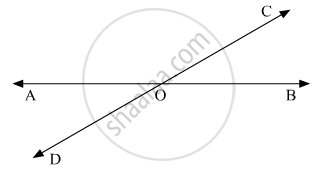# How Many Pairs of Adjacent Angles Are Formed When Two Lines Intersect in a Point? - Mathematics

How many pairs of adjacent angles are formed when two lines intersect in a point?

#### Solution

Let us draw the following diagram showing two lines AB and CD intersecting at a point O.We have the following pair of adjacent angles, so formed:

∠AOC and  ∠BOC

∠AOC and  ∠AOD

∠BOD and  ∠BOC

∠BOD and ∠AOD

Hence, in total four pair of adjacent angles are formed.

Concept: Concept of Parallel Lines
Is there an error in this question or solution?

#### APPEARS IN

RD Sharma Mathematics for Class 9
Chapter 10 Lines and Angles
Q 12 | Page 50